[home] [lexicon] [problems] [tests] [courses] [auxiliaries] [notes] [staff]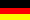Mathematics-Online lexicon:

# Eigenvalues and Eigenvectors of Symmetric Real Matrices

 A B C D E F G H I J K L M N O P Q R S T U V W X Y Z overview

Symmetric matrices overare normal. Thus they are diagonizable by a unitary transformation. With respect to the principal axis transformation of quadrics this property is of fundamental interest. Therefore we give a seperate summary of the properties of eigenvalues and eigenvectors of real symmetric matrices.

Letbe a real symmetric- matrix.

1.
All eigenvalues ofare real.
2.
Eigenvectors to different eigenvalues are orthogonal.
3.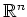has an orthonormal basis consisting of eigenvectors of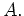4.
Letbe an orthonormal basis consisting of eigenvectors of. The matrix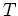, whose columns are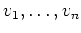, i.ehas the form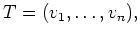is orthogonal, i.e..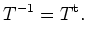5.
Letbe an orthonormal basis out of eigenvectors of, letand denote by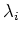the eigenvalue corresponding to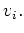Then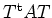is a diagonal matrix of the formNote that the eigenvaluesin the main diagonal are in the same ordering as the corresponding eigenvectors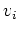as column vectors of